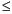Functions and CALL Routines

# PROBBETA Function

Returns the probability from a beta distribution.
 Category: Probability See: CDF Function

## Syntax

 PROBBETA(x,a,b)

### Arguments

x

is a numeric random variable.

 Range: 0x1
a

is a numeric shape parameter.

 Range: a > 0
b

is a numeric shape parameter.

 Range: b > 0

The PROBBETA function returns the probability that an observation from a beta distribution, with shape parameters a and b, is less than or equal to x.

SAS Statements Results
`x=probbeta(.2,3,4);`
`0.09888`

 Functions:Previous Page | Next Page | Top of Page